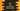# Java program to get the maximum number holder Student### Introduction :

In this tutorial, we will create and store some student’s details and find out the Student with the highest marks. We have two classes in this example. The first one is the ‘Student.java’ class and the second one is the ‘Main.java’ class. ‘Student.java’ will hold the information about a student. And, ‘Main.java’ is used to do other tasks like create a student, find the top student etc.

Let’s take a look into these classes first :

### Student.java class :

``````public class Student {
//1
//student name
private String studentName;

//student roll no
private int studentRollNo;

//marks of the student
private int studentMarks;

//age of the student
private int studentAge;

//2

/**
* Constructor to create a Student object
*
* @param name   : name
* @param rollNo : roll no
* @param marks  : marks
* @param age    : age
*/
public Student(String name, int rollNo, int marks, int age) {
this.studentName = name;
this.studentRollNo = rollNo;
this.studentMarks = marks;
this.studentAge = age;
}

//3

/**
* Get the name of the student
*
* @return : name of the student
*/
public String getStudentName() {
return studentName;
}

/**
* Get the roll no of the student
*
* @return : roll no of the student
*/
public int getStudentRollNo() {
return studentRollNo;
}

/**
* Get the marks of the student
*
* @return : Marks of the student
*/
public int getStudentMarks() {
return studentMarks;
}

/**
* Get the age of the student
*
* @return : Age of the student
*/
public int getStudentAge() {
return studentAge;
}
}``````
1. This class holds the name, roll-no, marks and age of a student. Each of these variables is ’private’, that means these variables cannot be accessed from outside of this class. You cannot access them by creating a new object of the class.

2. The constructor takes name, roll-no, marks and age as input. And it set these values to the private variables of this class.

3. Methods like ’getStudetName()’ is a ’Getter’ method. Using these methods, we get the value of the private variables. That means, to get the values of the private variables, we need to use these getter methods.

### Main.java class :

``````import java.util.ArrayList;

public class Main {

//8
/**
* Utility function for System.out.println
*
* @param message : string to print
*/
private static void println(String message) {
System.out.println(message);
}

/**
* main method
*
* @throws java.lang.Exception
*/
public static void main(String[] args) throws java.lang.Exception {

//1
ArrayList<Student> studentList = new ArrayList<>();

//2
//create objects of the student
Student danny = new Student("Danny", 2, 56, 12);
Student harry = new Student("Harry", 1, 46, 11);
Student ronny = new Student("Ronny", 3, 69, 10);
Student eddy = new Student("Eddy", 5, 26, 9);
Student calvin = new Student("Calvin", 4, 86, 13);

//3
//add all the students object to the ArrayList

//4
Student topStudent = null;
int maxMark = 0;

//5
//iterate through the list and record maximum marks and 'Student' object for that mark
for (int i = 0; i < studentList.size(); i++) { if (studentList.get(i).getStudentMarks() > maxMark) {
//6
topStudent = studentList.get(i);
maxMark = studentList.get(i).getStudentMarks();
}
}

//7
//print out the information of the top student

if (topStudent != null) {
println("Top Student Details : ");
println("Name : " + topStudent.getStudentName());
println("Age : " + topStudent.getStudentAge());
println("Roll No : " + topStudent.getStudentRollNo());
println("Marks : " + topStudent.getStudentMarks());
}

}

}``````

The commented numbers in the above program denote the step numbers below:

1. We created one ’ArrayList’ to save all values of different ’Student’ objects. The ArrayList takes values of type ’Student’. Name of the ’ArrayList’ is ’studentList‘.

2. We created five different types of ’Student’ objects for five different students. We used the constructors to set the values for the objects. Objects are saved in variables danny,harry,ronny,eddy and calvin.

3. Then, all ’Student’ objects are added to the ’ArrayList’ ’studentList‘.

4. Now, we need to find out the student with maximum marks among these objects. So, one variable to store the value of the top student is created as ’topStudent’. Also, to store the maximum mark, one variable is created as ’maxMark’ and set its value to zero, ‘0’.

5. Using one ’for’ loop to iterate through the list.

6. If the current ’Student’ object has marks greater than ’maxMark’ , set its value as ’maxMark’ and set the object value to ’topStudent‘.

7. After the loop is completed, print out the values of the ’Student’ objects. Use the ’getter’ methods to print the values.

### Output :

It will give the following output :

``````Top Student Details :
Name : Calvin
Age : 13
Roll No : 4
Marks : 86
``````